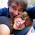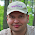### Django: Rotate Image with PIL usage.

Let's assume we have this simple model:

``````from django.db import models

class Image(models.Model):
image = models.FileField(upload_to="images/")```
```
`` ``
`Task is to take this simple image, rotate it and save instead of original.`
`Let's discover this way to do so:`
```
```
``````#views.py
from PIL import Image as PilImage
from models import Image

def rotate(request):
#getting instance of the model
item=Image.objects.get(pk=1)

#opening image for PIL to access
im = PilImage.open(image.image)

#rotating it by built in PIL command
rotated_image = im.rotate(270)

#saving rotated image instead of original. Overwriting is on.
rotated_image.save(item.image.file.name, overwrite=True)

return HttpResponse(str(image.image))```
```
```
```
`This pattern will work in most cases. Instead of rotate you can use any other PIL editing method, as for e.g. flip or sharpen the image... Have fun coding and please leave me a comment if you'll find this info useful. Thanks.`
```
```

### Comments

1.You spelled overwrite=True incorrectly.

1.Hek... Thanks.